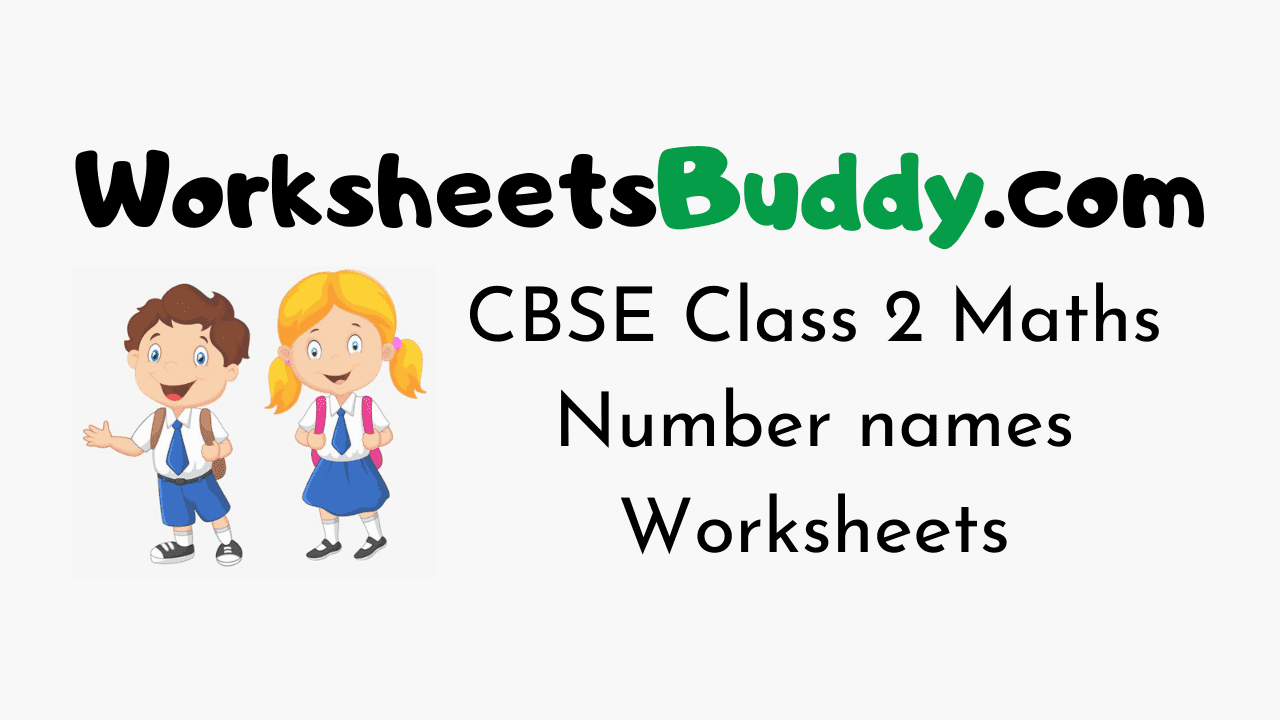# CBSE Class 2 Maths Number names Worksheets

CBSE Class 2 Maths Number names Worksheets – Worksheets have become an integral part and are the most engaging study materials for kids these days. The Standard 2 Maths Number names Worksheets will help your younger one to practice what they have learned in class. Number names Worksheets for Grade 2 will develop a curiosity in your kid to learn.

Maths Worksheets for Class 2 are a perfect combination of fun and learning. Find the most interesting and relevant puzzle activities for your kids here. In the Mathematics Grade 2 Number names Worksheets Puzzles will be in the form of crosswords, true or false, pictures, mazes, fill-ups, MCQs, objectives, questions and answers, and so on.

Mathematics Worksheets for Class 2 on Number names will help your kid remember what he/ she learned in school for a long time. Your Grade 2 kid will love to solve these engaging exercises and interesting collections of Puzzles.

### CBSE Class 2 Maths Number names Worksheets

1. Write the numerals of: Fifty Eight

2. Write the numerals of: Seventy Nine

3. Write the number names of: 87

4. Write the number names of: 42

5. Write the number names of: 34

6. Write the number names of: 71

7. Write the number names of: 90

8. Write the numerals of: Forty Two

9. Write the number names of: 20

10. Write the number names of: 55

11. Write the numerals of: Ninety Five

12. Write the numerals of: Twenty Three

13. Write the number names of: 76

14. Write the numerals of: Ninety Nine

15. Write the number names of: 58

16. Write the numerals of: Nineteen

17. Write the numerals of: Thirty

18. Write the numerals of: Sixty Five

19. Write the numerals of: Eighty One

20. Write the number names of: 93

CBSE Class 2 Maths Worksheet Answers

1. 58
2. 79
3. Eighty Seven
4. Forty Two
5. Thirty Four
6. Seventy One
7. Ninety
8. 42
9. Twenty
10. Fifty five
11. 95
12. 23
13. Seventy six
14. 99
15. Fifty Eight
16. 19
17. 30
18. 65
19. 81
20. Ninety Three### FAQs on Maths Number names Class 2 Worksheets

1. How does a child learn Mathematics Number names at Class 2 Level?

A child can learn Maths Number names at Class 2 Level through the worksheets prevailing on our page.

2. What are the topics in Maths Class 2?

Mathematics Class 2 will have topics like Number names etc.

3. Where can I get Mathematics Worksheets for Class 2 Number names for free?

You can get Maths Number names Worksheets Class 2 free of cost from our Worksheetsbuddy.com page. You can use them for your kid to get practice.

4. Are the CBSE Class 2 Number names Worksheets over here printable?

Yes, the CBSE Class 2 Number names Worksheets here are printable and you can download them easily.

Conclusion

We Hope the information prevailing on our page regarding CBSE Class 2 Mathematics Number names Worksheets has been useful to you. For more info feel free to reach us and we will assist you at the easiest possible. Keep in touch with our site for more information on Class Wise Worksheets for various subjects.